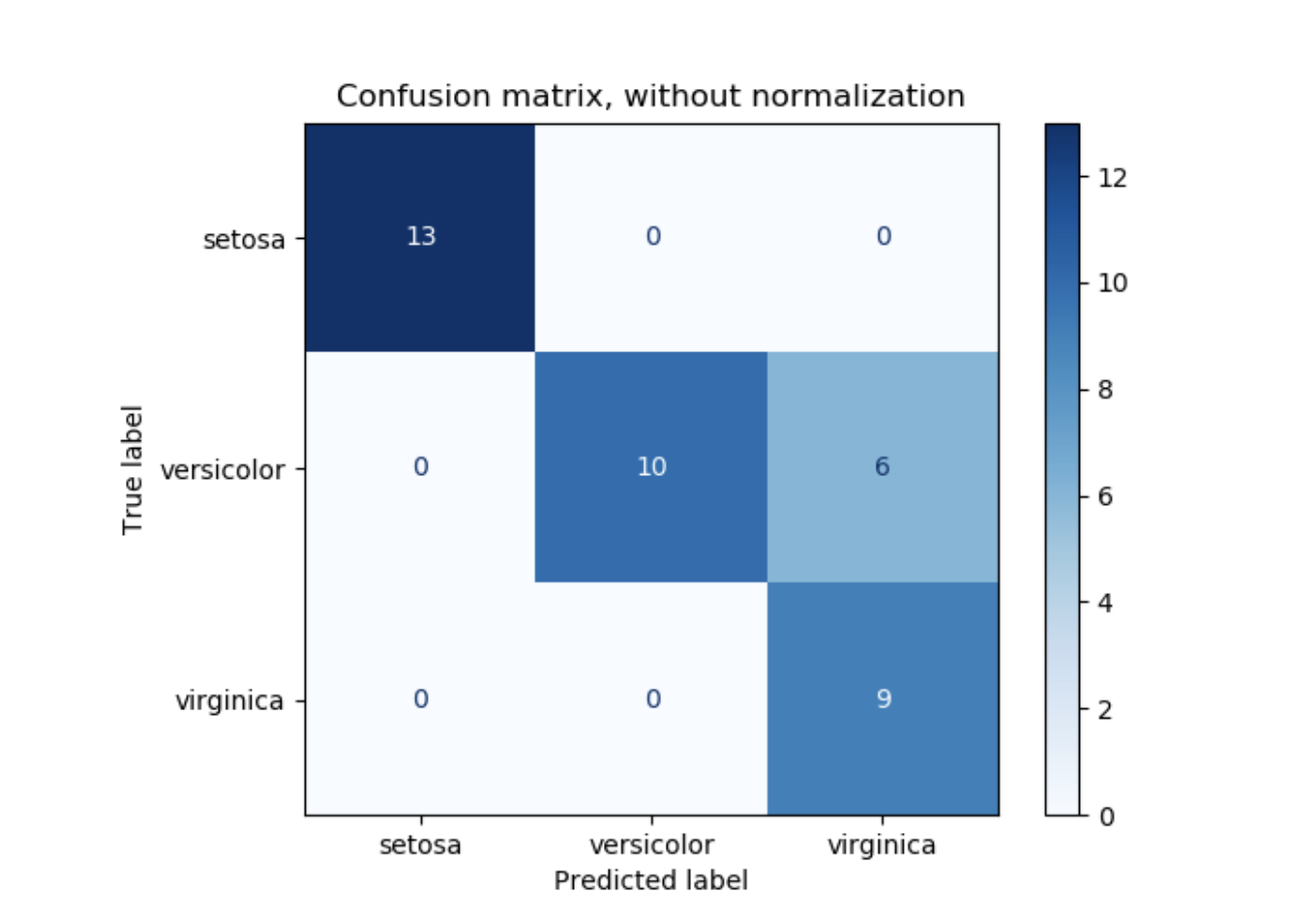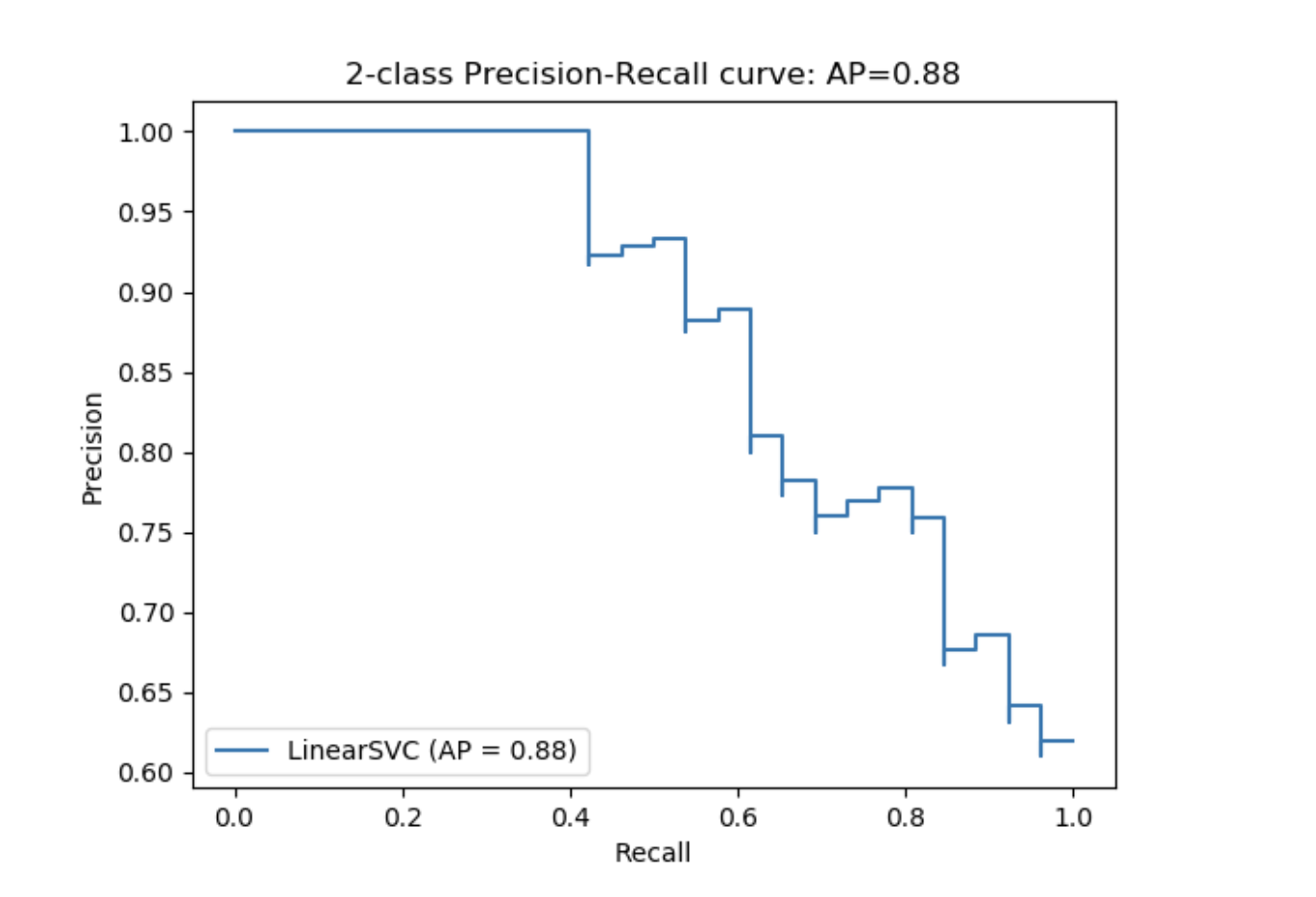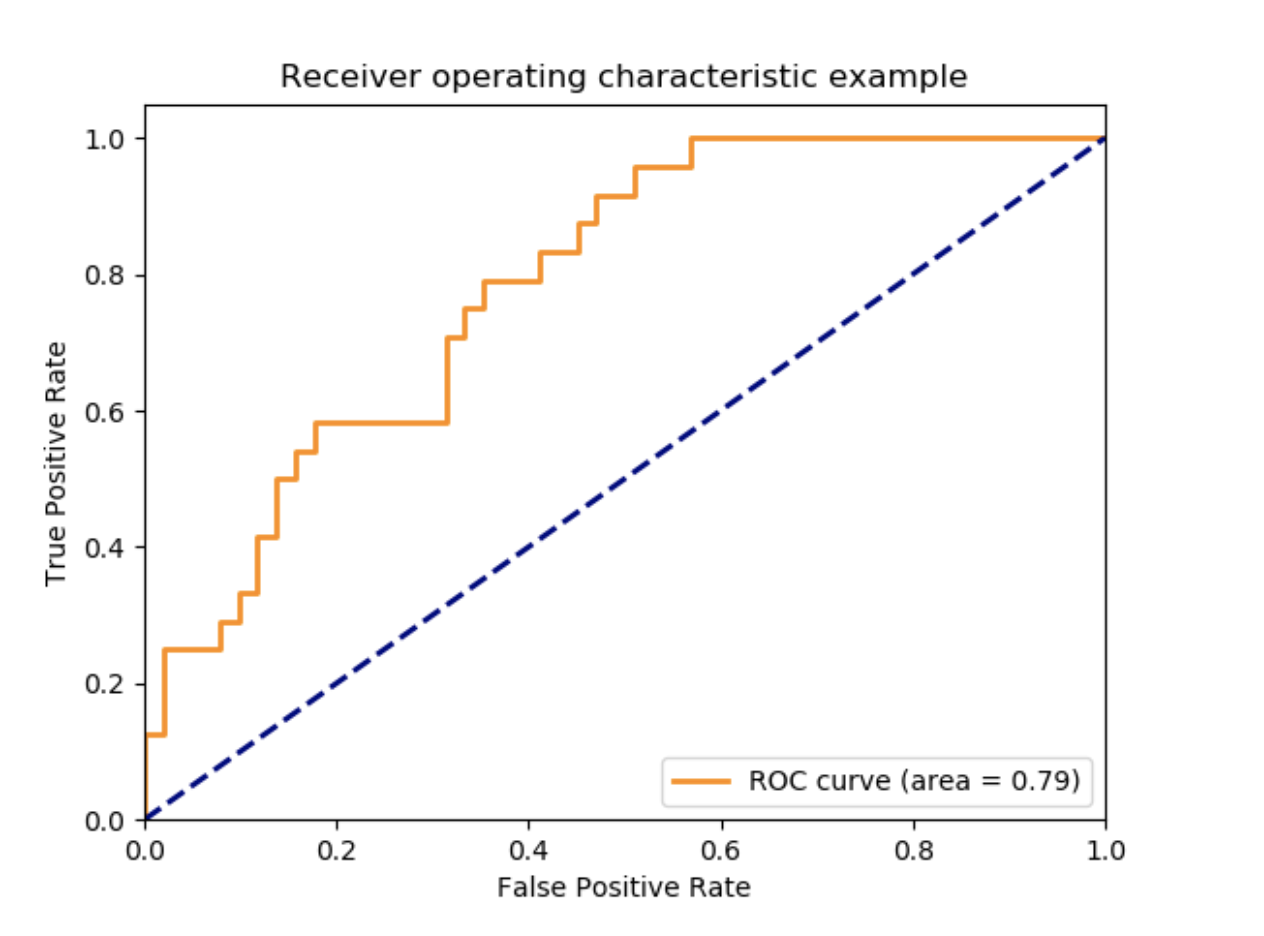# 3.3 指标和评分：量化预测的质量¶

3个不同的API可供评估模型预测质量：

## 3.3.1 评分参数：定义模型评估准则

### 3.3.1.1 常见场景：预定义值

"accuracy"（准确率） metrics.accuracy_score
"balanced_accuracy" （平衡准确率） metrics.balanced_accuracy_score
"average_precision"（平均精确率） metrics.average_precision_score
"neg_birer_score"（负福莱尔评分） metrics.brier_score_loss
"f1" metrics.f1_score 二分类
"f1_micro" metrics.f1_score 微平均
"f1_macro" metrics.f1_score 宏平均
"f1_weighted" metrics.f1_score 加权平均
"f1_samples" metrics.f1_score 多标签样本
"neg_log_loss" metrics.log_loss 要求predict_proba支持
"precision" 等 metrics_precision_score 后缀适用于“f1”
"recall" 等 metrics.recall_score 后缀适用于“f1”
"jaccard" 等 metrics.jaccard_score 后缀适用于“f1”
"roc_auc" metrics.roc_auc_score
"roc_acu_ovr" metrics.roc_auc_score
"roc_auc_ovo" metrics.roc_auc_score
"roc_auc_ovr_weighted" metrics.roc_auc_score
"roc_auc_ovo_weighted" metrics.roc_auc_score

"completeness_score" metrics.completeness_score
"fowlkes_mallows_score" metrics.fowlkes_mallows_score
"homogeneity_score" metrics.homogeneity_score
"mutual_info_score" metrics.mutual_info_score
"normalized_mutual_info_score" metrics.normalized_mutual_info_score
"v_measure_score" metrics.v_measure_score

"explained_variance" metrics.explained_variance_score
"max_error" metrics.max_error
"neg_mean_absolute_error" metrics.mean_absolute_error
"neg_root_mean_squared_error" metrics.mean_squared_error
"neg_mean_squared_log_error" metrics.mean_squared_log_error
"neg_median_absolute_error" metrics.median_absolute_error
"r2" metrics.r2_score
"neg_mean_poisson_deviance" metrics.mean_poisson_deviance
"neg_mean_gamma_deviance" metrics.mean_gamma_deviance

>>> from sklearn import svm, datasets>>> from sklearn.model_selection import cross_val_score>>> X, y = datasets.load_iris(return_X_y=True)>>> clf = svm.SVC(random_state=0)>>> cross_val_score(clf, X, y, cv=5, scoring='recall_macro')array([0.96..., 0.96..., 0.96..., 0.93..., 1.        ])>>> model = svm.SVC()>>> cross_val_score(model, X, y, cv=5, scoring='wrong_choice')Traceback (most recent call last):ValueError: 'wrong_choice' is not a valid scoring value. Use sorted(sklearn.metrics.SCORERS.keys()) to get va 

### 3.3.1.2 根据metric函数定义评分策略

sklearn.metrics模块公开一组简单函数，该函数可应用于测量给定真实值和预测值的预测误差：

• 以_score为结尾的函数，返回一个最大值，该值越大越好。
• 以_error或_loss为结尾的函数，返回一个最小值，该值越小越好。当使用make_scorer把它转变为评分对象时，设置greater_is_better参数为False（初始值是True；详见下面的函数描述）。

>>> from sklearn.metrics import fbeta_score, make_scorer>>> ftwo_scorer = make_scorer(fbeta_score, beta=2)>>> from sklearn.model_selection import GridSearchCV>>> from sklearn.svm import LinearSVC>>> grid = GridSearchCV(LinearSVC(), param_grid={'C': [1, 10]},...                     scoring=ftwo_scorer, cv=5) 

• 可以使用的python函数（下例中使用my_custom_loss_func）
• 是否python函数返回一个分数（初始值为greater_is_better=True）或者一个损失（分数）（greater_is_better=False）。如果一个损失函数，python函数的返回值是负值的评分对象，符合交叉验证的传统，评分越高，模型越好。
• 仅对于分类的指标：是否python函数要求提供连续决定确定性（needs_threshold=True）。初始值是False。
• 任何其它参数，如f1_score中的beta或labels。

>>> import numpy as np>>> def my_custom_loss_func(y_true, y_pred):...     diff = np.abs(y_true - y_pred).max()...     return np.log1p(diff)...>>> # score will negate the return value of my_custom_loss_func,>>> # which will be np.log(2), 0.693, given the values for X>>> # and y defined below.>>> score = make_scorer(my_custom_loss_func, greater_is_better=False)>>> X = [, ]>>> y = [0, 1]>>> from sklearn.dummy import DummyClassifier>>> clf = DummyClassifier(strategy='most_frequent', random_state=0)>>> clf = clf.fit(X, y)>>> my_custom_loss_func(clf.predict(X), y)0.69...>>> score(clf, X, y)-0.69... 

### 3.3.1.3 执行自定义评分对象

• 可以调用参数（estimator, X, y），estimator是需要被评估的模型，X是验证数据，y是X标签（应用于有监督的案例中）的真实值或者None（应用于非监督的案例中）。
• 它返回一个浮点型数值，该数值量化estimator对X关于y的预测质量。再强调一遍，越高的得分模型越好，所以如果得分是损失函数的返回值，那么该值应为负。

>>> from custom_scorer_module import custom_scoring_function >>> cross_val_score(model,...  X_train,...  y_train,...  scoring=make_scorer(custom_scoring_function, greater_is_better=False),...  cv=5,...  n_jobs=-1)  

### 3.3.1.4 使用多指标评估

Scikit-learn也允许在GridSearchCV，RandomizedSearchCV和cross_validate中评估多指标。

• 可迭代字符串指标::
>>> scoring = ['accuracy', 'precision'] 
• 以字典形式将评分器名称映射给评分函数::
>>> from sklearn.metrics import accuracy_score>>> from sklearn.metrics import make_scorer>>> scoring = {'accuracy': make_scorer(accuracy_score),...            'prec': 'precision'} 

>>> from sklearn.model_selection import cross_validate>>> from sklearn.metrics import confusion_matrix>>> # A sample toy binary classification dataset>>> X, y = datasets.make_classification(n_classes=2, random_state=0)>>> svm = LinearSVC(random_state=0)>>> def tn(y_true, y_pred): return confusion_matrix(y_true, y_pred)[0, 0]>>> def fp(y_true, y_pred): return confusion_matrix(y_true, y_pred)[0, 1]>>> def fn(y_true, y_pred): return confusion_matrix(y_true, y_pred)[1, 0]>>> def tp(y_true, y_pred): return confusion_matrix(y_true, y_pred)[1, 1]>>> scoring = {'tp': make_scorer(tp), 'tn': make_scorer(tn),...            'fp': make_scorer(fp), 'fn': make_scorer(fn)}>>> cv_results = cross_validate(svm.fit(X, y), X, y, cv=5, scoring=scoring)>>> # Getting the test set true positive scores>>> print(cv_results['test_tp'])[10  9  8  7  8]>>> # Getting the test set false negative scores>>> print(cv_results['test_fn'])[0 1 2 3 2] 

## 3.3.2 分类指标

sklearn.metrics模块执行各种损失函数，评分，及调用函数测量分类模型的表现。一些指标可能要求正类别的可能性（probability）估计，置信度值，或者二分类决策值。大多数执行允许计算每一个样本对评分的权重贡献，通过sample_weight参数实现。

precision_recall_curve(y_true, probs_pred, *) 根据不同的可能性阈值计算精确率-召回率
roc_curv(y_true, y_score, *[, pos_label, ...]) 计算Receiver operating characteristic(ROC)

balanced_accuracy_score(y_true, y_pred, *) 计算balanced准确率
cohen_kappa_score(y1, y2, *[, labels, ...]) Cohen's kappa：衡量注一致程度的统计
confusion_matrix(y_true, y_pred, *[, ...]) 计算混淆矩阵来评估分类模型的准确率
hinge_loss(y_true, pred_decision, *[, ...]) 平均hinge损失（非正规 non-regularized）
matthews_corrcoef(y_true, y_pred, *[, ...]) 计算曼哈顿相关系数（MCC）
roc_auc_score(y_true, y_score, *[, ...] 从预测分数中，计算ROC曲线的面积（ROC AUC）

accuracy_score(y_true, y_pred, *[, ...]) 分类模型的准确率得分
classification_report(y_true, y_pred, *[, ...]) 主要分类模型指标的文本报告
f1_score(y_true, y_pred, *[, labels, ...]) 计算F1评分，也被称为balanced F-score或者F-measure
fbeta_score(y_true, y_pred, *, beta[, ...]) 计算F-beta评分
hamming_loss(y_true, y_pred, *[, sample_weight]) 计算平均Hamming损失
jaccard_score(y_true, y_pred, *[, labels, ...]) Jaccard相似性系数得分
log_loss(y_true, y_pred, *[, eps, ...]) 对数损失，aka logistic损失或者交叉熵损失
multilabel_confusion_matrix(y_true, y_pred, *) 为每一个类或样本计算混淆矩阵
precision_recall_fscore_support(y_true, ...) 计算精确率，召回率，F-measure并且支持每一个类
precision_score(y_true, y_pred, *[, ...]) 计算精确率
recall_score(y_true, y_pred, *[, labels, ...]) 计算召回率
roc_auc_score(y_true, y_score, *[, ...]) 从预测分数中计算ROC曲线的面积
zero_one_loss(y_true, y_pred, *[, ...]) 0-1分类损失

average_precision_score(y_true, y_score, *) 从预测评分中计算平均精确率（AP）

### 3.3.2.1 从二分类到多分类和多标签

• "weighted" （权重）处理类的不均衡问题。按其在真实数据中的加权计算每个类的分数，并计算各二分类指标的平均值。
• "micro" （微）对于所有二分类指标（除非已分配样本权重）指定给每个样本类匹配相等的贡献。除了汇总每个类的指标，还要汇总除数和被除数，将每个类的各指标汇总成一个整体商（总被除数/总除数得出评估模型整体水平的商）。 微-平均方法更适合多标签分类中，包括多类别分类问题，但多数类会被忽略。
• "samples" （样本）仅应用于多标签问题。它不对子类进行计算，而是计算评估数据中每个样本真实类别和预测类别的指标。 最后返回它们的（sample_weight - weighted）均值。
• 设置参数 average = None 会返回每个类得分的数组。

### 3.3.2.2 准确率评分

accuracy_score函数计算accuracy（准确率），或者是个分数（初始值）或者是正确预测个数的计数（normalize=False）。

>>> import numpy as np>>> from sklearn.metrics import accuracy_score>>> y_pred = [0, 2, 1, 3]>>> y_true = [0, 1, 2, 3]>>> accuracy_score(y_true, y_pred)0.5>>> accuracy_score(y_true, y_pred, normalize=False)2 

>>> accuracy_score(np.array([[0, 1], [1, 1]]), np.ones((2, 2)))0.5 

### 3.3.2.3 平衡的准确率评分（Balanced accuracy score）

balanced_accuracy_score函数计算balanced accuracy，避免了对不平衡数据集进行夸大的性能估计。每个类别召回率的宏平均，或者等同于每个样本根据真实类别的比率（inverse prevalence）的权重，而计算的原始准确率。因此，对于平衡样本，平衡的准确率评分（Balanced accuracy score）与准确率相同。

**注意：**多分类的定义看起来是用于二分类指标的合理延伸，尽管在文献中未达成共识。

• 我们的定义：[Mosley2013], [Kelleher2015]和[Guyon2015], 其中[Guyon2015]适合调整的版本，以确保随机预测值为0，好的预测值为1..
• 类的均衡准确率在[Mosley2013]中描述：每个类别中精确率和召回率的最小值会被计算。将计算出的这些值进行平均，从而得到均衡的准确率。
• 均衡的准确率在[Urbanowicz2015]中被描述：每一个类别的灵敏度和特异性的均值会被计算，随后计算总的均值。

Guyon2015(1,2) I. Guyon, K. Bennett, G. Cawley, H.J. Escalante, S. Escalera, T.K. Ho, N. Macià, B. Ray, M. Saeed, A.R. Statnikov, E. Viegas, Design of the 2015 ChaLearn AutoML Challenge, IJCNN 2015.

Mosley2013(1,2) L. Mosley, A balanced approach to the multi-class imbalance problem, IJCV 2010.

Kelleher2015 John. D. Kelleher, Brian Mac Namee, Aoife D’Arcy, Fundamentals of Machine Learning for Predictive Data Analytics: Algorithms, Worked Examples, and Case Studies, 2015.

Urbanowicz2015Urbanowicz R.J., Moore, J.H. ExSTraCS 2.0: description and evaluation of a scalable learning classifier system, Evol. Intel. (2015) 8: 89.

### 3.3.2.4 Cohen's kappa

cohen_kappa_score函数计算Cohen's kappa统计值。此度量旨在比较不同标注的标签，而分类器和真实数据的比较。

Kappa score（参见文档字符串）是一个数值，介于-1和1之间。分数大于0.8会被认为是好的协议；小于0说明没有协议（等同于随机标签）。

Kappa score可被用于计算二分类或者多分类的问题，但不适用于多标签的问题（除手动计算每一个标签分数）和多于两个以上注释器的问题。

>>> from sklearn.metrics import cohen_kappa_score>>> y_true = [2, 0, 2, 2, 0, 1]>>> y_pred = [0, 0, 2, 2, 0, 2]>>> cohen_kappa_score(y_true, y_pred)0.4285714285714286 

### 3.3.2.5 混淆矩阵

confusion_matrix函数评估分类模型准确率，通过计算每一行相对应真实类别（维基百科或者其它资料可能使用不同的轴向）的混淆矩阵（confusion matrix）。

>>> from sklearn.metrics import confusion_matrix>>> y_true = [2, 0, 2, 2, 0, 1]>>> y_pred = [0, 0, 2, 2, 0, 2]>>> confusion_matrix(y_true, y_pred)array([[2, 0, 0],       [0, 0, 1],       [1, 0, 2]])  

plot_confusion_matrix可被用于展示混淆矩阵的可视化，在混淆矩阵的例子中，如下图所示：参数normalize允许报告比例而不仅是计数。混淆矩阵可以通过3种方式被规范化："pred", "true", 和"all"，这些参数将计算方式区分为按列求和，按行求和，或者整个矩阵求和。

>>> y_true = [0, 0, 0, 1, 1, 1, 1, 1]>>> y_pred = [0, 1, 0, 1, 0, 1, 0, 1]>>> confusion_matrix(y_true, y_pred, normalize='all')array([[0.25 , 0.125],       [0.25 , 0.375]]) 

>>> y_true = [0, 0, 0, 1, 1, 1, 1, 1]>>> y_pred = [0, 1, 0, 1, 0, 1, 0, 1]>>> tn, fp, fn, tp = confusion_matrix(y_true, y_pred).ravel()>>> tn, fp, fn, tp(2, 1, 2, 3) 

### 3.3.2.6 分类模型报告

classification_report函数输出一个文本报告，显示主要分类模型的评估指标。如下是一个自定义target_names和推断标签的例子：

>>> from sklearn.metrics import classification_report>>> y_true = [0, 1, 2, 2, 0]>>> y_pred = [0, 0, 2, 1, 0]>>> target_names = ['class 0', 'class 1', 'class 2']>>> print(classification_report(y_true, y_pred, target_names=target_names))              precision    recall  f1-score   support     class 0       0.67      1.00      0.80         2     class 1       0.00      0.00      0.00         1     class 2       1.00      0.50      0.67         2    accuracy                           0.60         5   macro avg       0.56      0.50      0.49         5weighted avg       0.67      0.60      0.59         5  

### 3.3.2.7 Hamming损失函数

Hamming_loss 计算平均的Hamming loss或者Hamming distance在两个样本的集合中。

>>> from sklearn.metrics import hamming_loss>>> y_pred = [1, 2, 3, 4]>>> y_true = [2, 2, 3, 4]>>> hamming_loss(y_true, y_pred)0.25 

>>> hamming_loss(np.array([[0, 1], [1, 1]]), np.zeros((2, 2)))0.75 

**注意：**在多分类的分类模型中，y_true和y_pred的Hamming损失函数与Hamming距离相一致，Hamming损失函数与零一损失函数（Zero one loss）相类似。但是零-一损失函数惩罚预测集合，预测集不与真实值集直接匹配，Hamming损失函数惩罚单个标签。因次，Hamming损失函数的上限是零一损失函数，它介于零和一之间，包括边界；预测真实标签合适的子集或者超子集会使Hamming损失函数界于零和一之间，不包括边界。

### 3.3.2.8 精确率，召回率和F-measures

F-measure测量）可被解释为精确率和召回率的权重调和平均数。测量值最高为1，最差为0。当时，是相等的，并且召回率和精确率是同等重要的。

precision_recall_curve从真实标签和评分（不同阈值设定下，分类器的评分）计算一个精确率-召回率曲线.

average_precision_score函数从预测分数中计算平均精确率（average precision（AP））。该值介于0和1之间，且越高越好。AP的定义如下：

average_precision_score(y_true, y_score,*) 从预测评分中计算平均精确率
f1_score(y_true, y_pred, *[labels, ...]) 计算F1评分，也被称为均衡的F-score或F-measure
fbeta_score(y_true, y_pred, *, beta[, ...]) 计算F-beta评分
precision_recall_curve(y_true, probas_pred, *) 计算在不同的阈值下精确率-召回率组合
precision_recall_fscore_support(y_true, ...) 计算精确率，召回率，F-measure，且支持对每一类的计算
precision_score(y_true, y_pred, *[, ...]) 计算精确率
recall_score(y_true, y_pred, *[, labels, ...]) 计算召回率例如：

[Manning2008] C.D. Manning, P.Raghavan, H. Schütze, Introduction to Information Retrieval, 2008.

[Everingham2010] M. Everingham, L.Van Gool, C.K.I. Williams, J. Winn, A. Zisserman, The Pascal Visual Object Classes (VOC) Challenge, IJCV 2010.

[Davis2006] J. Davis, M. Goadrich, The Relationship Between Precision-Recall and ROC Curves, ICML 2006.

[Flach2015] P.A. Flach, M. Kull, Precision-Recall-Gain Curves: PR Analysis Done Right, NIPS 2015.

#### 3.3.2.8.1 二分类分类模型

>>> from sklearn import metrics>>> y_pred = [0, 1, 0, 0]>>> y_true = [0, 1, 0, 1]>>> metrics.precision_score(y_true, y_pred)1.0>>> metrics.recall_score(y_true, y_pred)0.5>>> metrics.f1_score(y_true, y_pred)0.66...>>> metrics.fbeta_score(y_true, y_pred, beta=0.5)0.83...>>> metrics.fbeta_score(y_true, y_pred, beta=1)0.66...>>> metrics.fbeta_score(y_true, y_pred, beta=2)0.55...>>> metrics.precision_recall_fscore_support(y_true, y_pred, beta=0.5)(array([0.66..., 1.        ]), array([1. , 0.5]), array([0.71..., 0.83...]), array([2, 2]))>>> import numpy as np>>> from sklearn.metrics import precision_recall_curve>>> from sklearn.metrics import average_precision_score>>> y_true = np.array([0, 0, 1, 1])>>> y_scores = np.array([0.1, 0.4, 0.35, 0.8])>>> precision, recall, threshold = precision_recall_curve(y_true, y_scores)>>> precisionarray([0.66..., 0.5       , 1.        , 1.        ])>>> recallarray([1. , 0.5, 0.5, 0. ])>>> thresholdarray([0.35, 0.4 , 0.8 ])>>> average_precision_score(y_true, y_scores)0.83... 

#### 3.3.2.8.2 多分类和多标签分类模型

• y是预测值（样本，标签）组合的集合
• 是真实值（样本，标签）组合的集合
• L是标签的集合
• S是样本的集合
• y的样本s 的子集，例如：
• y的标签l的子集
• 同样地，均是的子集
• （在处理B=\emptyset时，公约有所不同；这个执行使用R(A, B): = 0，并且与P类似 ）

average Precision Recall F_beta
"micro"
"samples"
"macro"
"weighted"
None
>>> from sklearn import metrics
>>> y_true = [0, 1, 2, 0, 1, 2]
>>> y_pred = [0, 2, 1, 0, 0, 1]
>>> metrics.precision_score(y_true, y_pred, average='macro')
0.22...
>>> metrics.recall_score(y_true, y_pred, average='micro')
0.33...
>>> metrics.f1_score(y_true, y_pred, average='weighted')
0.26...
>>> metrics.fbeta_score(y_true, y_pred, average='macro', beta=0.5)
0.23...
>>> metrics.precision_recall_fscore_support(y_true, y_pred, beta=0.5, average=None)
(array([0.66..., 0.        , 0.        ]), array([1., 0., 0.]), array([0.71..., 0.        , 0.        ]), array([2, 2, 2]...))


>>> metrics.recall_score(y_true, y_pred, labels=[1, 2], average='micro')... # excluding 0, no labels were correctly recalled0.0 

>>> metrics.precision_score(y_true, y_pred, labels=[0, 1, 2, 3], average='macro')0.166... 

### 3.3.2.9 Jaccard相似性系数评分

jacquard_score函数计算Jaccard similarity coefficients（Jaccard相似性系数）的均值，也被称为Jaccard指数，在标签对的集合中。

i个样本的Jaccard相似性系数，真实标签和预测标签的定义如下：

jaccard_scoreprecision_recall_fscore_support的作用类似，应用于二分类标签，并且通过使用参数average可以扩展到多标签和多分类（详见above）。

>>> import numpy as np>>> from sklearn.metrics import jaccard_score>>> y_true = np.array([[0, 1, 1],...                    [1, 1, 0]])>>> y_pred = np.array([[1, 1, 1],...                    [1, 0, 0]])>>> jaccard_score(y_true, y_pred)0.6666... 

>>> jaccard_score(y_true, y_pred, average='samples')0.5833...>>> jaccard_score(y_true, y_pred, average='macro')0.6666...>>> jaccard_score(y_true, y_phred, average=None)array([0.5, 0.5, 1. ]) 

>>> y_pred = [0, 2, 1, 2]>>> y_true = [0, 1, 2, 2]>>> jaccard_score(y_true, y_pred, average=None)array([1. , 0. , 0.33...])>>> jaccard_score(y_true, y_pred, average='macro')0.44...>>> jaccard_score(y_true, y_pred, average='micro')0.33... 

### 3.3.2.10 Hinge loss

hinge_loss函数指通过使用hinge loss方法计算模型和数据之间的平均距离，它是一个单边指数，即仅考虑预测误差。（Hinge loss被用于最大化边际分类器，如支持向量机。）

>>> from sklearn import svm>>> from sklearn.metrics import hinge_loss>>> X = [, ]>>> y = [-1, 1]>>> est = svm.LinearSVC(random_state=0)>>> est.fit(X, y)LinearSVC(random_state=0)>>> pred_decision = est.decision_function([[-2], , [0.5]])>>> pred_decisionarray([-2.18...,  2.36...,  0.09...])>>> hinge_loss([-1, 1, 1], pred_decision)0.3... 

>>> X = np.array([, , , ])>>> Y = np.array([0, 1, 2, 3])>>> labels = np.array([0, 1, 2, 3])>>> est = svm.LinearSVC()>>> est.fit(X, Y)LinearSVC()>>> pred_decision = est.decision_function([[-1], , ])>>> y_true = [0, 2, 3]>>> hinge_loss(y_true, pred_decision, labels)0.56... 

### 3.3.2.11 对数损失函数（Log loss）

>>> from sklearn.metrics import log_loss>>> y_true = [0, 0, 1, 1]>>> y_pred = [[.9, .1], [.8, .2], [.3, .7], [.01, .99]]>>> log_loss(y_true, y_pred)0.1738... 

y_pred参数列表[.9, .1]中的第一个值表示第一个样本被标记为0的可能性为90%。对数损失函数是非负的。

### 3.3.2.12 马修斯相关系数

“马修斯相关系数被用于机器学习是作为测量二分类（两类）分类模型质量的评估。它考虑真正，假正，真负和假负，并且被认为是一个平衡值，可被用于类大小不均衡的数据。马修斯相关系数（MCC）本质上是一个介于-1和+1之间的相关系数值。如果系数值为+1，则表示预测非常好，0表示一个平均随机的预测，-1表示预测相反。在统计学中也被称作phi系数。”

• 是类别k真实发生的次数，
• 是类别k预测的次数，
• 是正确预测的总样本个数，
• 是总样本的个数。

>>> from sklearn.metrics import matthews_corrcoef>>> y_true = [+1, +1, +1, -1]>>> y_pred = [+1, -1, +1, +1]>>> matthews_corrcoef(y_true, y_pred)-0.33... 

### 3.3.2.13 多标签混合矩阵

multilabel_confusion_matrix函数计算class-wise（初始值）或者sample-wise（sample wise = True）多标签混淆矩阵来评估分类模型的准确率。如果是多标签，多标签混淆矩阵（multilabel_confusion_matrix）也被认为是多分类数据。这种转变被普遍用于使用二分类分类模型指标（例如精确率，召回率等。）评估多分类问题。

>>> import numpy as np>>> from sklearn.metrics import multilabel_confusion_matrix>>> y_true = np.array([[1, 0, 1],...                    [0, 1, 0]])>>> y_pred = np.array([[1, 0, 0],...                    [0, 1, 1]])>>> multilabel_confusion_matrix(y_true, y_pred)array([[[1, 0],        [0, 1]],       [[1, 0],        [0, 1]],       [[0, 1],        [1, 0]]]) 

>>> multilabel_confusion_matrix(y_true, y_pred, samplewise=True)array([[[1, 0],        [1, 1]],<BLANKLINE>       [[1, 1],        [0, 1]]]) 

>>> y_true = ["cat", "ant", "cat", "cat", "ant", "bird"]>>> y_pred = ["ant", "ant", "cat", "cat", "ant", "cat"]>>> multilabel_confusion_matrix(y_true, y_pred,...                             labels=["ant", "bird", "cat"])array([[[3, 1],        [0, 2]],       [[5, 0],        [1, 0]],       [[2, 1],        [1, 2]]]) 

>>> y_true = np.array([[0, 0, 1],...                    [0, 1, 0],...                    [1, 1, 0]])>>> y_pred = np.array([[0, 1, 0],...                    [0, 0, 1],...                    [1, 1, 0]])>>> mcm = multilabel_confusion_matrix(y_true, y_pred)>>> tn = mcm[:, 0, 0]>>> tp = mcm[:, 1, 1]>>> fn = mcm[:, 1, 0]>>> fp = mcm[:, 0, 1]>>> tp / (tp + fn)array([1. , 0.5, 0. ]) 

>>> tn / (tn + fp)array([1. , 0. , 0.5]) 

>>> fp / (fp + tn)array([0. , 1. , 0.5]) 

>>> fn / (fn + tp)array([0. , 0.5, 1. ]) 

### 3.3.2.14 Receiver operating characteristic (ROC)

“receiver operating characteristic (ROC)，或简称ROC 曲线， 是一个图，它解释二分类分类器系统随着不同阈值变化的表现。用来绘制在各种阈值的设置中，真正数占总正样本的比率（TPR = 真正率（true positive rate））与假正数占总负样本的比率（FPR = 假正率（false positive rate））之间的关系。TPR也被认为是敏感度，和FPR（1-敏感度或者真负率）。”

>>> import numpy as np>>> from sklearn.metrics import roc_curve>>> y = np.array([1, 1, 2, 2])>>> scores = np.array([0.1, 0.4, 0.35, 0.8])>>> fpr, tpr, thresholds = roc_curve(y, scores, pos_label=2)>>> fprarray([0. , 0. , 0.5, 0.5, 1. ])>>> tprarray([0. , 0.5, 0.5, 1. , 1. ])>>> thresholdsarray([1.8 , 0.8 , 0.4 , 0.35, 0.1 ])roc_auc_score函数计算ROC曲线的面积，被称为AUC或者AUROC。通过计算ROC曲线的面积，整个曲线的信息被总结为一个数字。详见Wikipedia article on AUC

>>> import numpy as np>>> from sklearn.metrics import roc_auc_score>>> y_true = np.array([0, 0, 1, 1])>>> y_scores = np.array([0.1, 0.4, 0.35, 0.8])>>> roc_auc_score(y_true, y_scores)0.75 

One-vs-one Algorithm: 计算所有可能类组合的AUC的均值。[HT2001]定义一个多类别AUC指标均匀权重：

[HT2001]多分类AUC指标可以延伸到用prevalence进行加权：

**One-vs-rest Algorithm: **计算每个类别的AUC而不是剩余的[PD2000]。该算法在功能上与多标签的例子相同。通过设置"ovr"给参数multiclass来使用该算法。正如OvO, OvR支持两种均值策略：“macro”[F2006]和"weighted"[F2001]。

HT2001(1,2) Hand, D.J. and Till, R.J., (2001). A simple generalisation of the area under the ROC curve for multiple class classification problems. Machine learning, 45(2), pp.171-186.

[FC2009]Ferri, Cèsar & Hernandez-Orallo, Jose & Modroiu, R. (2009). An Experimental Comparison of Performance Measures for Classification. Pattern Recognition Letters. 30. 27-38.

[PD2000]Provost, F., Domingos, P. (2000). Well-trained PETs: Improving probability estimation trees (Section 6.2), CeDER Working Paper #IS-00-04, Stern School of Business, New York University.

[F2006]Fawcett, T., 2006. An introduction to ROC analysis. Pattern Recognition Letters, 27(8), pp. 861-874.

[F2001]Fawcett, T., 2001. Using rule sets to maximize ROC performance In Data Mining, 2001. Proceedings IEEE International Conference, pp. 131-138.

### 3.3.2.15 Zeor one loss

zero_one_loss函数是通过来计算0-1分类模型的损失函数（）的求和或者均值。初始情况下，函数会将整个数据标准化。为了得到的求和，设置normalize=False。

>>> from sklearn.metrics import zero_one_loss>>> y_pred = [1, 2, 3, 4]>>> y_true = [2, 2, 3, 4]>>> zero_one_loss(y_true, y_pred)0.25>>> zero_one_loss(y_true, y_pred, normalize=False)1 

>>> zero_one_loss(np.array([[0, 1], [1, 1]]), np.ones((2, 2)))0.5>>> zero_one_loss(np.array([[0, 1], [1, 1]]), np.ones((2, 2)),  normalize=False)1 

“Brier score是一个合适的评分函数，它测量概率预测的准确率。它适用于预测必须将该率分配给一组相互排斥的离散结果的任务。”

brier分数损失也是一个介于0和1之间的数，评分越低（均方差很小），预测越准确。它被看作是测量一个可能性预测集合的“标尺”。

>>> import numpy as np>>> from sklearn.metrics import brier_score_loss>>> y_true = np.array([0, 1, 1, 0])>>> y_true_categorical = np.array(["spam", "ham", "ham", "spam"])>>> y_prob = np.array([0.1, 0.9, 0.8, 0.4])>>> y_pred = np.array([0, 1, 1, 0])>>> brier_score_loss(y_true, y_prob)0.055>>> brier_score_loss(y_true, 1 - y_prob, pos_label=0)0.055>>> brier_score_loss(y_true_categorical, y_prob, pos_label="ham")0.055>>> brier_score_loss(y_true, y_prob > 0.5)0.0 

• 参见 Probability calibration of classifiers 是一个使用Brier score损失来校准分类器的可能性。

## 3.3.3 多标签排序指标

### 3.3.3.1 覆盖误差

coverage_error函数计算必须包含在最中预测的标签的平均数量，以预测所有真实的标签。如果想知道高分数标签的个数，需要可以预测不缺少任何真实标签的平均值。因此，该指标的最佳值是真实标签的平均值。

**注意：**这里实现的得分比在Tsoumakas et al., 2010文献中提供的计算方式大1.这个延伸是为了解决一些例子中不包含真实标签的退化现象。